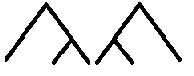## Thursday, April 6, 2017

### Detail #336: Modelling Restrictions on Compounds

Languages with compounds can have restrictions on what compounds are permitted. Describing such a system of restrictions in some depth could be a nice way of getting an impressive grammar done. Let us consider some ways of 'modelling' such a system. There's a difference between modelling and exhaustively describing, in some sense.

Giving an exhaustive description is possible for a conlanger: we inform the reader how it works and since we're the creators, our fiat holds. However, this might be somewhat uninteresting. Models are interesting in that they attempt to catch what happens, but might simplify some stuff and therefore be mistaken about things as well.

Given the natural scope of a language - spoken over generations, spoken by lots of speakers in varying relations with one another (all the way from family to have never ever interacted due to not even living in the same centuries nor even geographically all that close) it is likely for there to be a lot of variation in some parts of the language, and thus a model makes a lot of sense: it'll be wrong some of the time, but it'll catch the main traits of the system.

So, let's consider compounding and how we could model restrictions on it. First, we can recognize two types of edges of a compound: the left edge and the right edge. We can imagine a compound that does not permit any added morpheme to the left, and the same goes for the right. We call these 'right-saturated' and 'left-saturated' compounds. A compound that is saturated at both edges is simply saturated.

Another thing about modelling is that it'd be good if it also helped parse the compounds. Thus, a good model should tell us whether an element in a compound is a left-branch or a right-branch by looking at the word. It should even, probably, tell us whether two neighbouring elements are only "superficial" neighbours.Left and right-branching

This gives our model some actual usefulness beyond its 'descriptive' power. Now we come to the nitty-gritty stuff. We of course want to have some way of quantifying whether a word accepts compounding. Let's simply use numbers for this - we could put it in a range [0, 1], where 1 is 'accepts compounding' and '0' is 'saturated' and values inbetween are probabilistic estimates as to how likely it is to accept compounding. So, we have, for any word, two values left and right ∈ [0, 1]. I'll write left and right as a single vector C = (x, y), where x is the left and y is the right edge. Subscript text comes in four varieties: full words represent themselves. Thus CDonauSchiff is the compound of Donau and Schiff. One-letter capital variables represent an arbitrary word. Small letters

Let us take two words, Donau and Schiff. These have associated vectors CDonau and CSchiff. The resulting Donauschiff too has the associated vector CDonauSchiff, which is a product of the vectors of the two elements. The interesting thing, of course, is the function that takes  CDonau and CSchiff and produces CDonauSchiff. It should be clear that order is relevant - we wouldn't expect Schiffdonau and Donauschiff to have the same properties. A very simple model would do something like this:
CEF = (El, Fr), where l and r as subscripts mark "left edge value" and "right edge value".
In such a model, the property at the edges carry on down. However, there's no a priori reason why ABl = Al and ABr = Br. In other words, there's no reason why a compound's edges should have the same compounding-properties as the element that occupies those edges - shoemaker needs not have the same left-edge property as shoe and right-edge property as maker - in fact, we'd sort of maybe expect, in English, that shoemaker would be more similar at the left edge to maker than to shoe (but not maybe entirely so). The compound is a new word, possibly a word of a different word-class (with regards to at least one of its parts), and thus it seems unjustified to expect the compoundability to be conserved at edges.

Thus we probably want a more detailed idea of what compounds are permitted - we might want both Cl and Cr to be vectors for different types of lexemes: verbs, proper nouns, nouns of different classes, adjectives of different kinds, etc. We might even want to go further: probabilities for specific inflected forms, probabilities for 'heavy' words vs. 'light' words, measured by their nested structure, etc.

Amyways, my next step in modelling this would be to come up with some kind of 'average' probability per word class pair, e.g. adjective-noun 75%, inanimate_noun-transitive_verb 80%. Once this is done, I'd make a weighted graph, where nodes are types of words, and directed edges are the probability of a word of one type compounding before a word of some other type. Self-cycles may exist.

Next, each lexical item in the conlang's lexicon would be given a run where a randomizer decides whether it'll accept a certain word-type as prefix or suffix with the probability given by that graph. The probabilities for the new word's edges would be based on some way of measuring 'saturation', which again creates a new thing we might need: a saturated word does not permit more suffixes, and this may happen even if there are non-zero probabilities going on for some level of the compound at the edges.

I am not going to present any algorithm for this now, this is basically an early rambling intended to come up with something.